last updated: 2022-12-26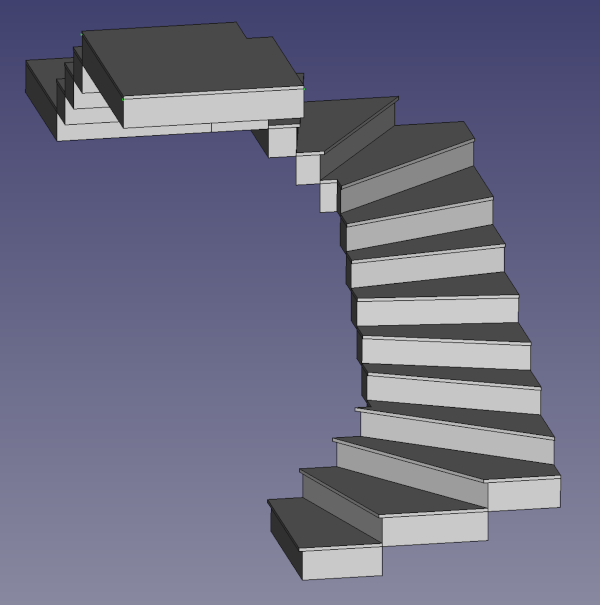### Intro

I wanted to draw stairs for a house, an found the Macro Half turn stairs from Berner. It did not work as expected with FreeCAD version 0.20, and so I tried to understand the macro and reprogrammed it.

Fortunately FreeCAD macros use python and so this was not so hard. I wanted to use the Spreadsheet workbench from FreeCAD.

The Macro can draw stairs from a given floor height going up or down with a flooring if needed. If the stairs go up, we can even add a flooring overhang.I

### The sketch

The first step was to draw a sketch from the stairs: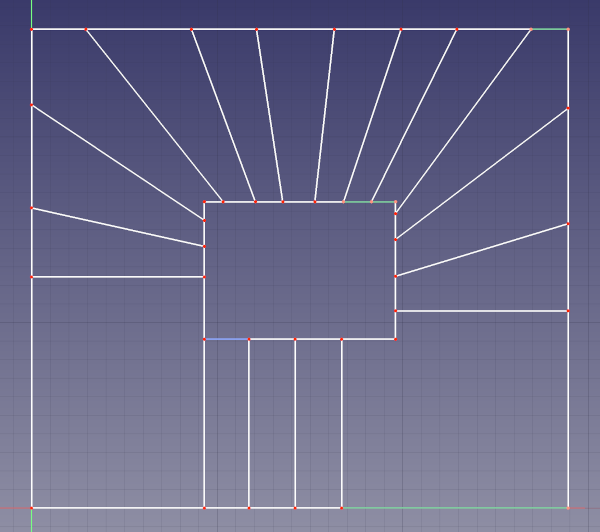There is no need for the sketch to be fully constraint. You can add only the important constraints that you e.g. measured on a real stair.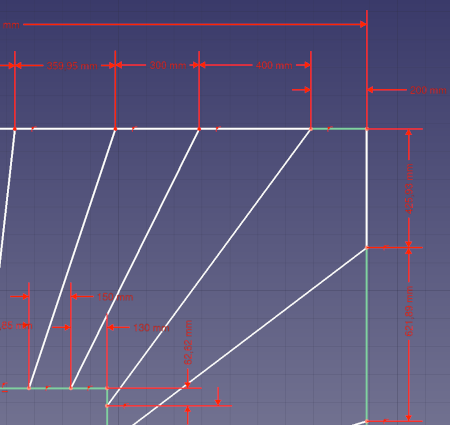The next step is to add the x,y-coordinates of the step vertices ass reference with the contrain horizontal and vertical distance tools: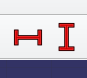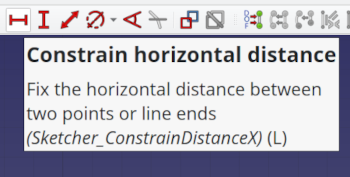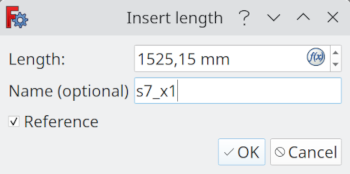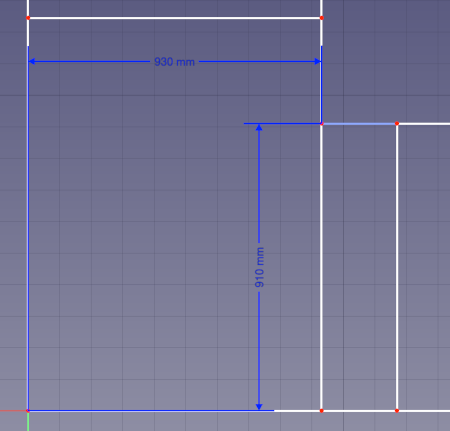Now we can add a spreadsheet to our file and add the reference to the cells. The header line will be ignored in the macro. The first cell of a row contains the step number, followed by x,y,z tupels for each x,y vertex of the step. The z cell of the tuple contains 0 as these values are calculated by the macro. The row can contain up to 7 tupel. The tupels must follow a clock or counterclock path of a step.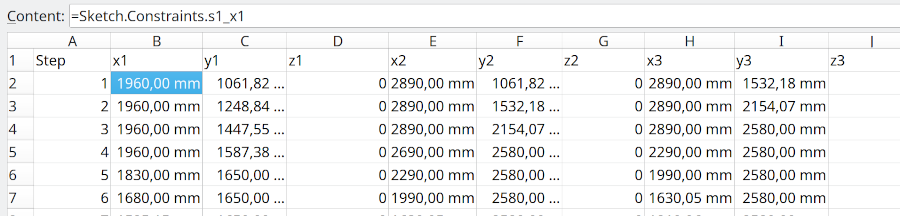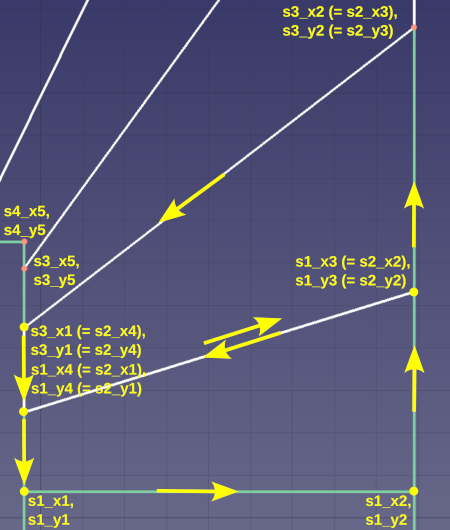The x,y vertices can be addressed in the spreadsheet with `=Sketch.Constraints.name`. After this lengthy work we get a spreadsheet with all the points, that can be exported as CSV-file. We save the file in the macro folder of FreeCAD (in Kubuntu: `/home/user/.local/share/FreeCAD/Macro/`)

Freecad uses as standard a tabulator as delimiter. One could use the spreadsheet directly in the macro, but I thought it more flexible to export a file and import it in the macro.

### The CSV file

Here my CSV file in the Kate editor: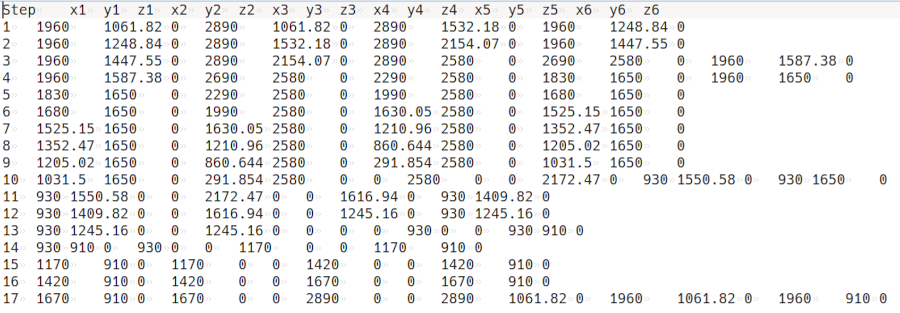### The macro

The macro calculates the step height from the floor height. We can walk the stairs up or down, and we can extrude the steps under their base.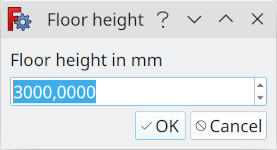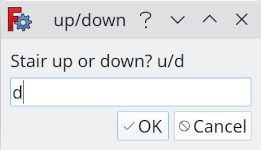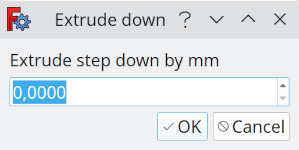We can also add a flooring with a flooring overhang (the overhang only for stairs up!).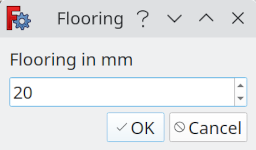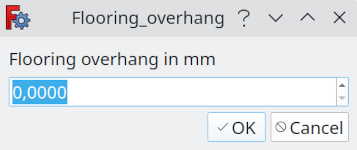Here an example for a stair going down with a flooring of 20 mm: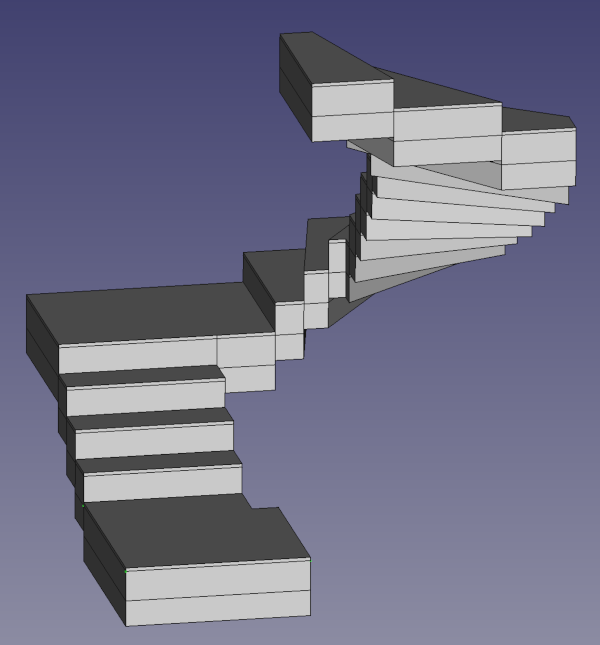A displacement to the origin (x,y,z) can be added.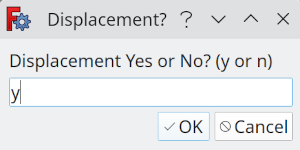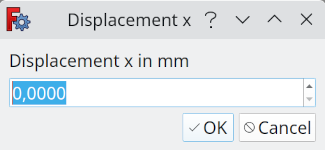The macro renames the wire and extrude labels.
If the constant `ARCH` in the the macro is `True`, an Arch stair component is created by adding all the step to the first step. If the constant `ARCH` in the the macro is `False`, everything is grouped under a group named `stairs`: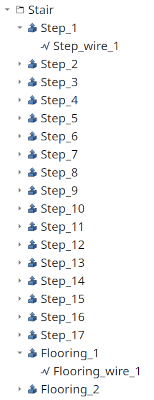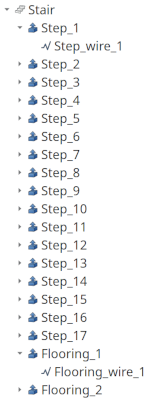Now here is the code, that you can also find on github:

``````    # -*- coding: utf-8 -*-
#
#
# Macro based on half_turn_stairs macro from Berner
#
# Author: weigu.lu based on work from Berner and Forum
#
# This macro creates a stair from tupels of x,y,z.
# The 3-7 tupels (e.g. x1,y1,z1,x2,y2,z2,x3,y3,z3,x4,y4,z4) are used
# to create a closed polyline (draftwire, dwire) for every step_height.
# The polyline is extruded. The step height is calculated from
# the floor height (step_height=total height/number of step_heights).
#
# A sample file with parameters is in stairs.csv. This file must
# be in the macro folder. The value seperator is the tabulator.
# One step per line. The first line is ignored.
# The first cell contains the step number.
#
# Example for first stair:
# 1 0.0 -240.0 0.0 0.0 0.0 0.0 1000.0 0.0 0.0 1000.0 -240.0 0.0
#
# A flooring with overhang can be created for stairs going up.
# For stairs going down, the overhang is not implemented.
# With ARCH = True an ARCH component called is created
# ARCH = False creates a group of parts
# A displacement to the original coordinates can be added

from __future__ import unicode_literals
from PySide import QtGui
import csv
import math

DATASET_FILENAME = "stairs.csv"
DATASET_DELIMITER = '\t'
ARCH = True # False creates a group of parts, True an ARCH component

### FUNCTIONS ###
def get_data_from_csv_file(csv_filename, delimiter):
""" Get data from CSV file """
data = []
with open(csv_filename, newline='') as csvfile:
data.append(line)
return data

def get_data_from_gui():
""" Get data from GUI """
ok = True
total_height, ok = QtGui.QInputDialog.getDouble(QtGui.QWidget(), "Floor height",
"Floor height in mm", 2890, 0, 4000, 4)
if (not ok):
return 0,0,0,0,0,0,(0,0,0)
up_down, ok = QtGui.QInputDialog.getText(None, "Up or Down?", "Stair up or down? (u or d)",text="u")
if (not ok):# or (up_down != 'u') or (up_down != 'd'):
return 0,0,0,0,0,0,(0,0,0)
extrude_down, ok = QtGui.QInputDialog.getDouble(QtGui.QWidget(), "Extrude down",
"Extrude step down by mm", 100, 0, 1000, 4)
if (not ok):
return 0,0,0,0,0,0,(0,0,0)
flooring, ok = QtGui.QInputDialog.getDouble(QtGui.QWidget(), "Flooring",
"Flooring in mm", 20, 0, 1000, 4)
if (not ok):
return 0,0,0,0,0,0,(0,0,0)
if flooring:
flooring_overhang, ok = QtGui.QInputDialog.getDouble(QtGui.QWidget(), "Flooring_overhang",
"Flooring overhang in mm", 0, 0, 1000, 4)
if (not ok):
return 0,0,0,0,0,0,(0,0,0)
else:
flooring_overhang = 0
displacement, ok = QtGui.QInputDialog.getText(None, "Displacement?",
"Displacement Yes or No? (y or n)",text="n")
if (not ok):
return 0,0,0,0,0,0,(0,0,0)
if displacement == 'y':
displace_x, ok = QtGui.QInputDialog.getDouble(QtGui.QWidget(), "Displacement x",
"Displacement x in mm", 0, 0, 100000, 4)
if (not ok):
return 0,0,0,0,0,0,(0,0,0)
displace_y, ok = QtGui.QInputDialog.getDouble(QtGui.QWidget(), "Displacement y",
"Displacement y in mm", 0, 0, 100000, 4)
if (not ok):
return 0,0,0,0,0,0,(0,0,0)
displace_z, ok = QtGui.QInputDialog.getDouble(QtGui.QWidget(), "Displacement z",
"Displacement z in mm", 0, 0, 100000, 4)
if (not ok):
return 0,0,0,0,0,0,(0,0,0)
else:
displace__x = displace_y = displace_z = 0
return ok, total_height, up_down, extrude_down, flooring, flooring_overhang, \
(displace_x, displace_y, displace_z)

def create_wire(wire_name,vector_list, placement= False,closed=False,support=None,face=None):
""" Create a wire (polyline) from a vector list and give it a name ; from forum """
Draft._Wire(wire_object)
wire_object.Points = vector_list
wire_object.Closed = closed
wire_object.Support = support
if face != None:
wire_object.MakeFace = face
if placement: wire_object.Placement.Base = placement
if Gui:
Draft._ViewProviderWire(wire_object.ViewObject)
Draft.formatObject(wire_object)
Draft.select(wire_object)

def extrude_wire(wire_name, extrude_name, extrude_fwd, extrude_rev):
""" Extrude a wire and choose a name for the extrusion """
active_document_name = App.ActiveDocument.Name
Extr_Obj.Base = App.getDocument(active_document_name).getObject(wire_name)
Extr_Obj.DirMode = str("Custom")
Extr_Obj.Dir = App.Vector(0.000000000000000, 0.000000000000000, 1.000000000000000)
Extr_Obj.LengthFwd = extrude_fwd
Extr_Obj.LengthRev = extrude_rev
Extr_Obj.Solid = True
Extr_Obj.Reversed = False
Extr_Obj.Symmetric = False
Extr_Obj.TaperAngle = 0.000000000000000
Extr_Obj.TaperAngleRev = 0.000000000000000

def create_step_wires(data, name, step_nr, up_down, nr_of_steps, step_height, offset, displace):
""" Create FreeCAD wires from data """
num_words = len(data[step_nr])
num_tupel = num_words // 3
#App.Console.PrintMessage("Step nr: " + str(step_nr) + " Number of words: " + str(num_words) +
#                                      " Number of tupels: " + str(num_tupel) + "\n")
if num_words == (num_tupel * 3) and num_words > 0:
vector = [0,0,0,0,0,0,0]
for i in range(0, num_tupel):
if up_down == 'u':
data[step_nr][i*3+2] = (step_nr-1) * step_height + offset
else:
data[step_nr][i*3+2] = (nr_of_steps-(step_nr)) * step_height + offset
float(data[step_nr][i*3+2]))
#App.Console.PrintMessage(str(vector) + "\n")
if num_tupel == 3:
vectorlist = [vector,vector,vector]
elif num_tupel == 4:
vectorlist = [vector,vector,vector,vector]
elif num_tupel == 5:
vectorlist = [vector,vector,vector,vector,vector]
elif num_tupel == 6:
vectorlist = [vector,vector,vector,vector,vector,vector]
elif num_tupel == 7:
vectorlist = [vector,vector,vector,vector,vector,vector,vector]
else:
App.Console.PrintMessage("more than 7 vertices per step (xy) not supported," +
" expand the macro :)\n")
else:
App.Console.PrintMessage("Error in data file line nr " + str(step_nr)  +
". No multiple of 3 words\n")
wire_name = name + str(step_nr)
create_wire(wire_name, vectorlist, placement, closed=True)

def extrude_step_wires(step_nr, step_height, extrude_down):
""" Extrude step wires """
wire_name = "Step_wire_" + str(step_nr)
extrude_name = "Step_" + str(step_nr)
extrude_wire(wire_name, extrude_name, step_height, extrude_down)

def extrude_flooring_wires(step_nr, flooring):
""" Extrude flooring wires """
wire_name = "Flooring_wire_" + str(step_nr)
extrude_name = "Flooring_" + str(step_nr)
extrude_wire(wire_name, extrude_name, flooring, 0)

def create_flooring_data(data, nr_of_steps, step_nr, up_down, flooring_overhang):
""" Create flooring data and flooring overhang (only up) """
#App.Console.PrintMessage(str(data[step_nr]) + "\n") # Write to console
x1_next = 0,
x2_next = 0
y1_next = 0
y2_next = 0
x1_prev = 0
x2_prev = 0
y1_prev = 0
y2_prev = 0
x1 = float(data[step_nr])
y1 = float(data[step_nr])
x2 = float(data[step_nr])
y2 = float(data[step_nr])
if step_nr != nr_of_steps: # last step has no next step
x1_next = float(data[step_nr+1])
y1_next = float(data[step_nr+1])
x2_next = float(data[step_nr+1])
y2_next = float(data[step_nr+1])
if step_nr != 1: # first step has no prev step
x1_prev = float(data[step_nr-1])
y1_prev = float(data[step_nr-1])
x2_prev = float(data[step_nr-1])
y2_prev = float(data[step_nr-1])
angle = math.atan2((y2-y1),(x2-x1)) # for degrees: *180/math.pi
#App.Console.PrintMessage("Step: " + str(step_nr) + " Angle: " +  str(angle) + "\n" )
cornerflag = 0  # we get a problem a step point equals a corner point
if x1 == x1_next: # vertical
cornerflag = cornerflag + 1
data[step_nr] = y1-(flooring_overhang/math.cos(angle))
if x2 == x2_next: # vertical
cornerflag = cornerflag + 1
data[step_nr] = y2-(flooring_overhang/math.cos(angle))
if y1 == y1_next: # horizontal
cornerflag = cornerflag + 1
data[step_nr] = x1+(flooring_overhang/math.sin(angle))
if y2 == y2_next: # horizontal
data[step_nr] = x2+(flooring_overhang/math.sin(angle))
cornerflag = cornerflag + 1
if cornerflag < 2:
if x1 == x1_prev and  x1 != x1_next:
data[step_nr] = y1-(flooring_overhang/math.cos(angle))
if x2 == x2_prev and  x2 != x2_next:
data[step_nr] = y2-(flooring_overhang/math.cos(angle))
if y1 == y1_prev and  y1 != y1_next:
data[step_nr] = x1+(flooring_overhang/math.sin(angle))
if y2 == y2_prev and  y2 != y2_next:
data[step_nr] = x2+(flooring_overhang/math.sin(angle))
#App.Console.PrintMessage(str(data[step_nr]) + "\n\n") # Write to console
return data

for step_nr in range(1, nr_of_steps+1):
label_name = name + str(step_nr)

for step_nr in range(1, nr_of_steps+1):
label_name = name + str(step_nr)
if label_name != "Step_1":

def macro_body():
### INIT DATA AND GET DATA ###
p=FreeCAD.ParamGet("User parameter:BaseApp/Preferences/Macro") # Get location of user macros
path = p.GetString("MacroPath")
dataset_filename = path + "/" + DATASET_FILENAME     # path and name of file.txt
ok, total_height, up_down, extrude_down, flooring, flooring_overhang, displace = get_data_from_gui()
if not ok: # stop macro if "Cancel" pressed in GUI
return
data = get_data_from_csv_file(dataset_filename, DATASET_DELIMITER)
### MAIN ###
nr_of_steps = int(data[-1]) #get the first value of last line
if flooring:
total_height = total_height-flooring
step_height = float(total_height/nr_of_steps)  # Height of one step
#App.Console.PrintMessage("Nr_of_steps: " + str(nr_of_steps) + "  Stepheight: " +
#                                                   str(step_height) + "\n")
# create wires
for step_nr in range(1,nr_of_steps+1):
data[step_nr].pop(0) #remove first value (step number)
create_step_wires(data, "Step_wire_", step_nr, up_down, nr_of_steps, step_height,0,displace)
# extrude
for step_nr in range(1, nr_of_steps+1):
extrude_step_wires(step_nr, step_height, extrude_down)
if ARCH:
Stair_obj = Arch.makeStairs(App.ActiveDocument.getObjectsByLabel("Step_1"),
length=None, width=None, height=None, steps=None, name = "Stair")
else:
# flooring
if flooring:
for step_nr in range(1, nr_of_steps+1):
if up_down == 'd': # flooring with overhang works only for stairs going up!
data = create_flooring_data(data,  nr_of_steps, step_nr, up_down, 0)
else:
data = create_flooring_data(data,  nr_of_steps, step_nr, up_down, flooring_overhang)
create_step_wires(data, "Flooring_wire_", step_nr, up_down, nr_of_steps,
step_height, step_height, displace)
for step_nr in range(1, nr_of_steps+1):
extrude_flooring_wires(step_nr, flooring)
if ARCH: